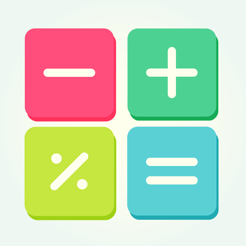## Screenshots

•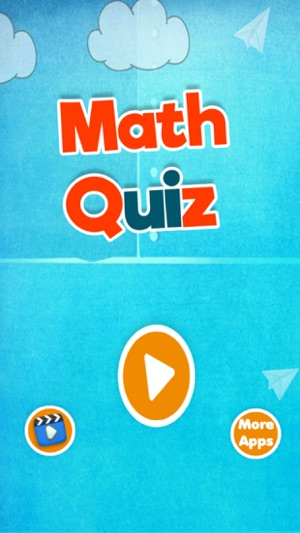•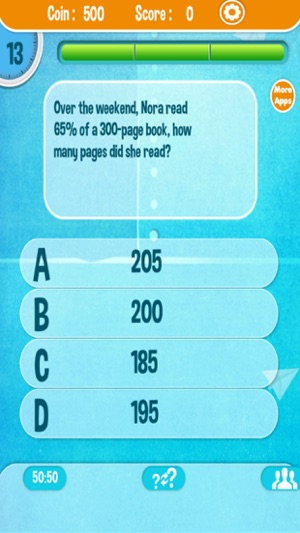••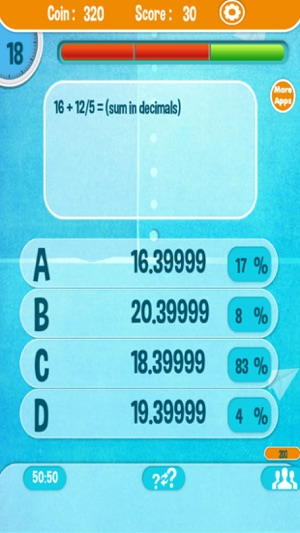•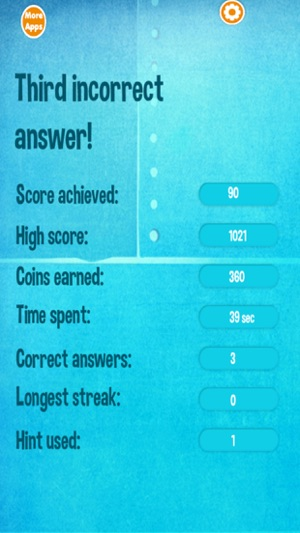## Description

For all Math lovers, introducing a brand new Maths Quiz & Trivia game! A quiz game that could easily become your favorite math game! Amazing math trivia quiz to help you become a real math solver and learn the most important concepts of maths.

Maths Quiz with lots of Brain teasers, to help you do some math practice and solve tricky math puzzles! Tons of quiz trivia questions to discover maths.

Features -
- Choose one of 4 multiple choice answers
- Various levels of difficulty with endless questions and answers
- If you get stuck in some question, you can ask for 3 types of help:
1) 50:50 – remove two incorrect answers;
2) Switch your question with another one;

Are you searching for a math quiz game to practice basic math operations? Or an app that offers mathematical, general aptitude, reasoning and logical puzzles to speed up your maths skills for your next competitive exam? Are you a math expert searching for a brain game to give your brain a workout? You've come to the right place! Math Puzzles Game is a free math quiz game with great set of challenging math puzzles, quizzes and various math tricks to improve your mental math.

Brainy Maths lets you play math quizzes of basic arithmetic operations like addition, subtraction, multiplication, division or statistics like average, mean, median or complex math concepts like sequence and series. Solve challenging math puzzles and be Cool Math Master!

## Information

Seller
divya mehta
Size
24.2 MB
Category
Games
Compatibility

Requires iOS 8.1 or later. Compatible with iPhone, iPad, and iPod touch.

Languages

English

Age Rating
4+
Price
Free

## Supports

•